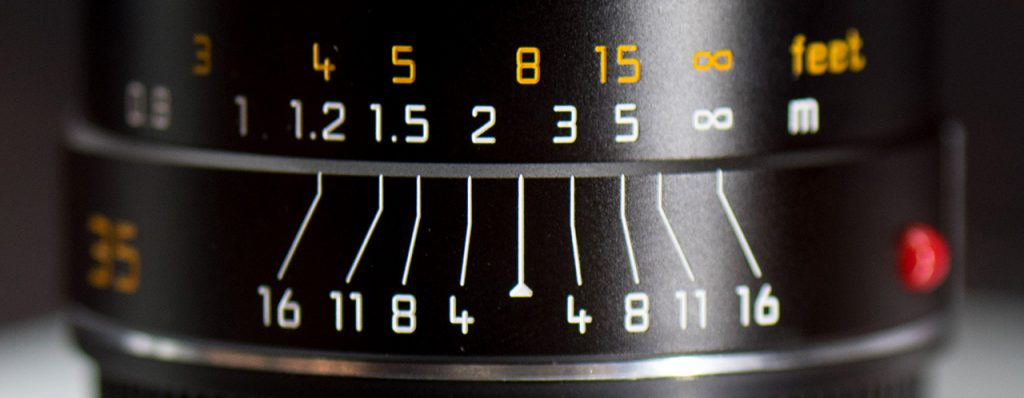# 10 Hyperfocal Distance

In many cases, especially in landscape photography, we are interested to get an image which is sharp from near the camera all the way to the horizon. Common wisdom is to focus at the shortest possible distance such that the depth of field still stretches to infinity, i.e.

 gfar = ∞ (H1)

where gfar denotes the far limit. The corresponding focus distance is known as the hyperfocal distance (literally super focus distance, which maximizes the scene in focus).

According to equation (D8b), the far limit can be derived from the subject distance g, the focal length f of the lens, the f-stop A and the circle of confusion c as follows:

 gfar = g f2 / (f2 – A c (g – f)) (H2)

Since the focal length is finite, the expression on the right can only become infinite if either the subject distance is infinite or the denominator is zero. The latter is used to calculate the hyperfocal distance ghyper:

 f2 – A c (ghyper – f) = 0 (H3)

Solving for ghyper gives

 ghyper = f2 / (A c) + f (H4)

Note that ghyper is an subject distance, measured from the lens. With equation (F3), the corresponding focus distance dhyper can be derived as

 dhyper = f2 / (A c) + 2 f + A c (H5)

This is the formula used for the hyperfocal distance calculator.

## 10.1 Approximation

For all practical values, everything but the first term in equation (H5) can be neglected, thus we get the following approximation

 dhyper ≈ f2 / (A c) (H6)

## 10.2 Near limits

Since we have established that we can focus at the hyperfocal distance or at infinity (or in fact anywhere in between) with the far limit still reaching infinity, it is interesting to compare the near limits of these approaches. These can be calculated using equation (D8a).

• For a lens focused at the hyperfocal distance, the near limit is approximately given by
 gnear ≈ f2 / (2 A c) (H7)

In other words, the depth of field already begins at half the hyperfocal distance.

• For a lens focused at infinity, we get the approximation
 gnear ≈ f2 / (A c) (H8)

In this case, the depth of field starts at the hyperfocal distance (which is quite a coincidence).

For example, for a focal length of 50 mm, f-stop 8 and a circle of confusion of 0.033 mm, we get a hyperfocal distance of 9.6 m (31 ft) and a near limit of 4.8 m (16 ft). For the lens focused at infinity, the near limit is at 9.5 m (31 ft), somewhat further away from the camera.

## 10.3 Practical considerations

With today’s autofocus lenses, manually focusing at some numerical distance seems not only a bit outdated, but also rather impractical. Even more so, as manual focusing is often operated by wire, many of these lenses don’t provide a distance scale any more. As a workaround, if you use the autofocus on some subject a few meters beyond the calculated hyperfocal distance, you should be on the safe side.

The situation is a lot easier with many manual focus lenses which provide a depth of field scale, such as the Leica Summarit lens shown below. Left and right of the marker for the focus distance, additional markers for the various f-stops indicate the respective extension of the depth of field. To focus at the hyperfocal distance, simply turn the focus ring such that the infinity symbol of the distance scale is aligned with the right DOF marker for the f-stop that you are using (in this case 16). The hyperfocal distance is at about 2.4 m (8 ft), with the depth of field extending from 1.2 m (4 ft) to infinity. This ease of use has certainly contributed significantly to the popularity of the hyperfocal distance.So problem solved … or is it?